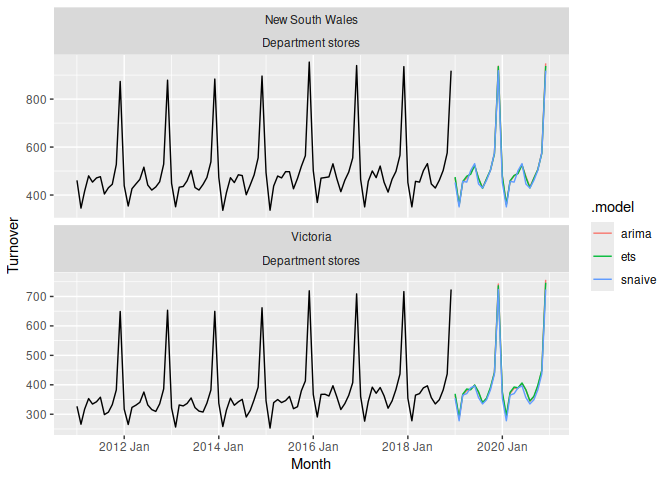The R package fable provides a collection of commonly used univariate and multivariate time series forecasting models including exponential smoothing via state space models and automatic ARIMA modelling. These models work within the fable framework, which provides the tools to evaluate, visualise, and combine models in a workflow consistent with the tidyverse.

Installation

The v0.1.0 pre-release can be installed using:

install.packages("fable", repos = "https://tidyverts.org/")

You can install the development version from GitHub

Installing this software requires a compiler

Example

library(fable)
library(tsibble)
library(tsibbledata)
library(lubridate)
library(dplyr)
aus_retail %>%
filter(
State %in% c("New South Wales", "Victoria"),
Industry == "Department stores"
) %>%
model(
ets = ETS(box_cox(Turnover, 0.3)),
arima = ARIMA(log(Turnover)),
snaive = SNAIVE(Turnover)
) %>%
forecast(h = "2 years") %>%
autoplot(filter(aus_retail, year(Month) > 2010), level = NULL)Getting help

• Questions about forecasting can be asked on Cross Validated.

• Common questions about the fable package are often found on Stack Overflow. You can use this to ask for help if the question isn’t already answered. A minimally reproducible example that describes your issue is the best way to ask for help!